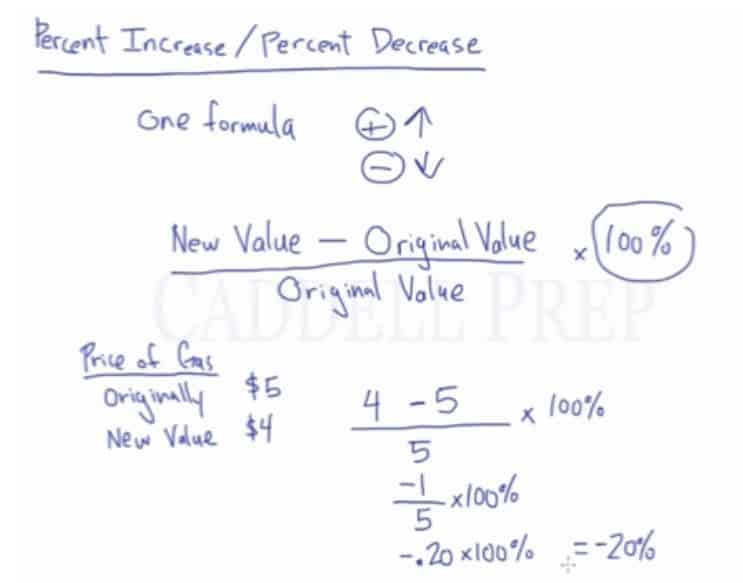In this video, we will be learning how to determine percent change. After you finish this lesson, view all of our Pre-Algebra lessons and practice problems.

Percent Change Formula:$\dfrac{New Value - Original Value}{Original Value}\cdot100\%=Percent Change$

For example:

The price of gas was originally $5. The new price for gas is$4. What is the percent change?$\dfrac{New Value - Original Value}{Original Value}\cdot100\%=Percent Change$$\dfrac{(4) - (5)}{(5)}\cdot100\%=Percent Change\leftarrow$ Substitute the givens$\dfrac{(4) - (5)}{(5)}\cdot100\%=Percent Change\leftarrow$ Simplify$\dfrac{-1}{5}\cdot100\%=Percent Change$$-20\%=Percent Change$

## Examples of Percent Change

### Example 1

The staff at a company went from 40 to 29 employees. What is the percent decrease in staff?$\dfrac{New Value - Original Value}{Original Value}\cdot100\%=Percent Change$

First, substitute the given$\dfrac{(29) - (40)}{(40)}\cdot100\%=Percent Change$

Then, simplify$\dfrac{-11}{40}\cdot100\%=Percent Change$$-27.5\%=Percent Change$

### Example 2

Jessica works in a candystore for $8.00 per hour. If her pay is increased to$14.00, then what is her percent increase in pay?$\dfrac{New Value - Original Value}{Original Value}\cdot100\%=Percent Change$

First, substitute the given$\dfrac{(14) - (8)}{(8)}\cdot100\%=Percent Change$

Then, simplify$\dfrac{6}{8}\cdot100\%=Percent Change$$75\%=Percent Change$

## Video-Lesson Transcript

In this video, we will be learning how to determine percent change.

Let’s go through percent increase and percent decrease.

We will use just one formula to compute the percent change:$\dfrac{New Value - Original Value}{Original Value} \times 100\%$

For example:

The price of gas was originally$\5$. The price went down to$\4$. What is the percent change?

Let’s use the formula.$\dfrac{4 - 5}{5} \times 100\%$

Which will be$\dfrac{(- 1)}{5} \times 100\%$

Then divide$(- 1)$ by$5$ the answer is$(- 0.20)$$(- 0.20) \times 100\% = (-20\%)$

We have a$20\%$ decrease.Let’s have another one.

The price of gas was originally$\4$. The price went down to$\5$. What is the percent change?

Let’s use the formula and substitute all the given values.$\dfrac{5 - 4}{4} \times 100\%$$\dfrac{1}{4} \times 100\%$$0.25 \times 100\%$

We’ll get$25\%$

Since the answer is positive, it means it’s an increase.

Just a review, the percent change formula is$\dfrac{New Value - Original Value}{Original Value} \times 100\%$